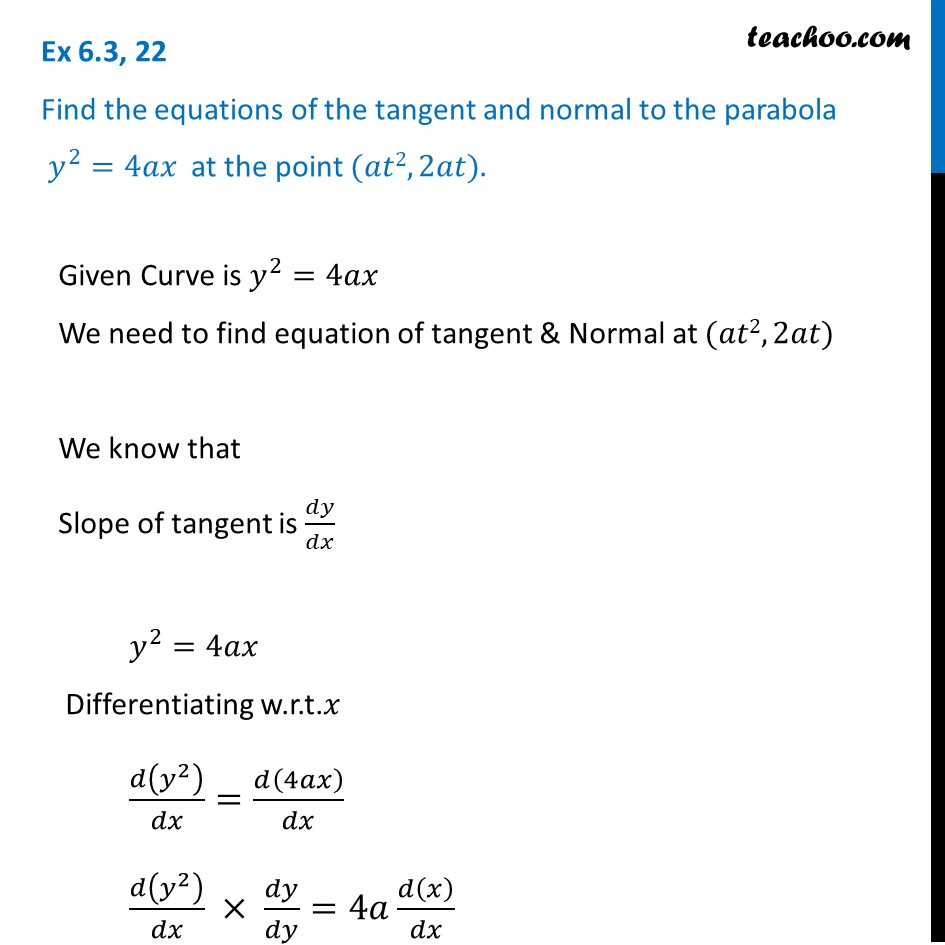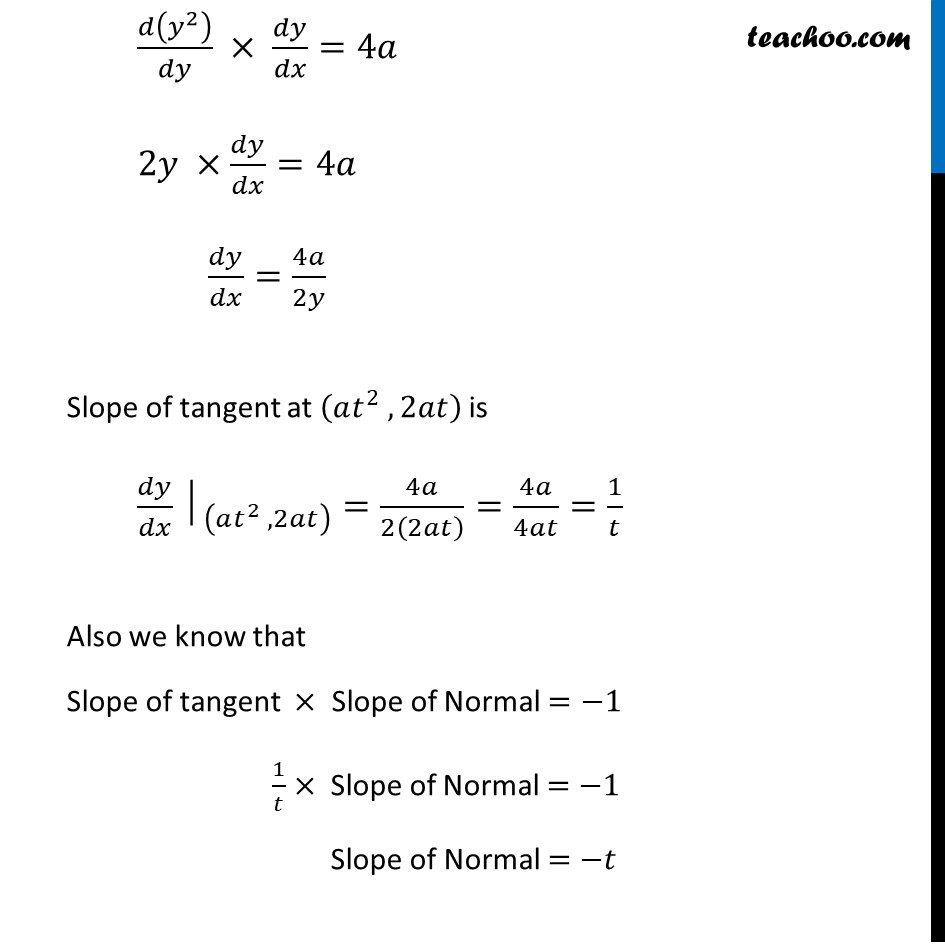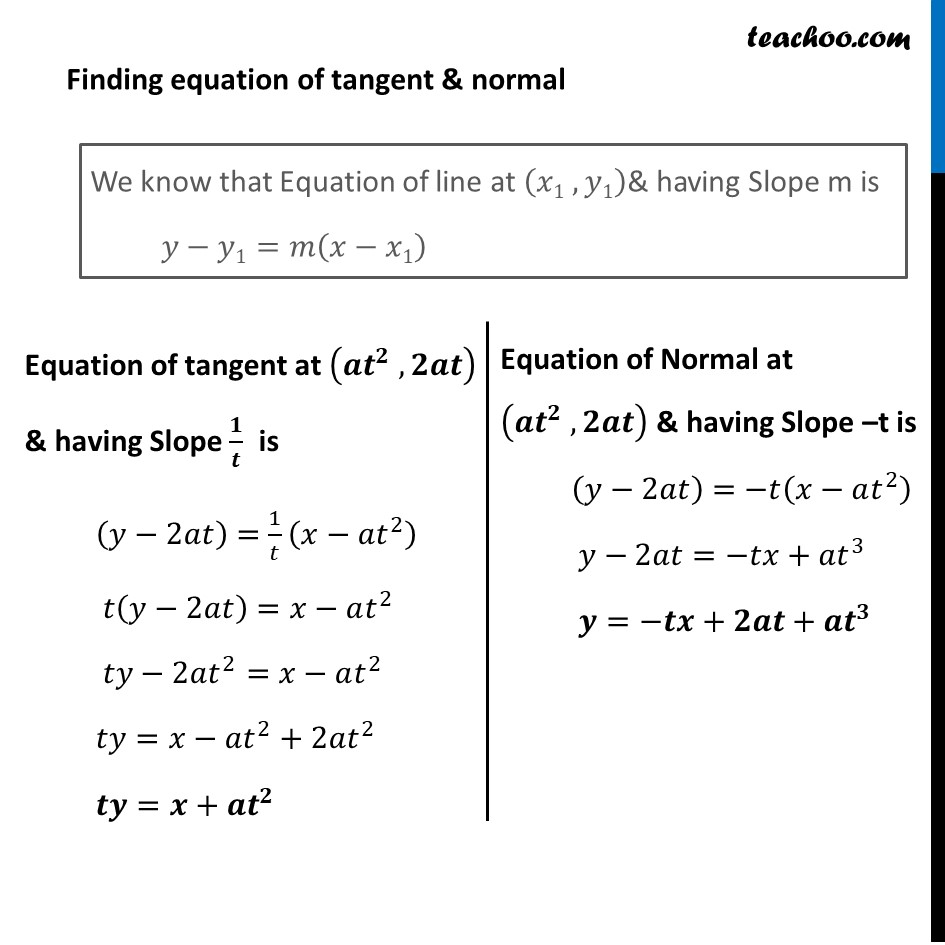Finding equation of tangent/normal when point and curve is given

Chapter 6 Class 12 Application of Derivatives
Concept wiseLearn in your speed, with individual attention - Teachoo Maths 1-on-1 Class

### Transcript

Question 22 Find the equations of the tangent and normal to the parabola 𝑦^2=4𝑎𝑥 at the point (𝑎𝑡2, 2𝑎𝑡).Given Curve is 𝑦^2=4𝑎𝑥 We need to find equation of tangent & Normal at (𝑎𝑡2, 2𝑎𝑡) We know that Slope of tangent is 𝑑𝑦/𝑑𝑥 𝑦^2=4𝑎𝑥 Differentiating w.r.t.𝑥 𝑑(𝑦^2 )/𝑑𝑥=𝑑(4𝑎𝑥)/𝑑𝑥 𝑑(𝑦^2 )/𝑑𝑥 × 𝑑𝑦/𝑑𝑦=4𝑎 𝑑(𝑥)/𝑑𝑥 𝑑(𝑦^2 )/𝑑𝑦 × 𝑑𝑦/𝑑𝑥=4𝑎 2𝑦 ×𝑑𝑦/𝑑𝑥=4𝑎 𝑑𝑦/𝑑𝑥=4𝑎/2𝑦 Slope of tangent at (𝑎𝑡^2 , 2𝑎𝑡) is 〖𝑑𝑦/𝑑𝑥│〗_((𝑎𝑡^2 , 2𝑎𝑡) )=4𝑎/2(2𝑎𝑡) =4𝑎/4𝑎𝑡=1/𝑡 Also we know that Slope of tangent × Slope of Normal =−1 1/𝑡× Slope of Normal =−1 Slope of Normal =−𝑡 Finding equation of tangent & normal We know that Equation of line at (𝑥1 , 𝑦1)& having Slope m is 𝑦−𝑦1=𝑚(𝑥−𝑥1) Equation of tangent at (𝒂𝒕^𝟐 , 𝟐𝒂𝒕) & having Slope 𝟏/𝒕 is (𝑦−2𝑎𝑡)=1/𝑡 (𝑥−𝑎𝑡^2 ) 𝑡(𝑦−2𝑎𝑡)=𝑥−𝑎𝑡^2 𝑡𝑦−2𝑎𝑡^2=𝑥−𝑎𝑡^2 𝑡𝑦=𝑥−𝑎𝑡^2+2𝑎𝑡^2 𝒕𝒚=𝒙+𝒂𝒕^𝟐 Equation of Normal at (𝒂𝒕^𝟐 , 𝟐𝒂𝒕) & having Slope –t is (𝑦−2𝑎𝑡)=−𝑡(𝑥−𝑎𝑡^2 ) 𝑦−2𝑎𝑡=−𝑡𝑥+𝑎𝑡^3 𝒚=−𝒕𝒙+𝟐𝒂𝒕+𝒂𝒕^𝟑